Equation of a Circle homework Help at TutorEye

# Best Homework Help For Equation of a Circle

## Top Questions

t schedule 3 people for the day my % scheduled is 78.57% My question is how did the computer come up with the 78.57%?
View More

I would love to get a written solution. I did the other proof tree that you can see in the attaached files. So its supposed to look like that Thank You.
View More

xtbook. i really need in in half an hour
View More

## Equation of a Circle

The equation of a circle maps the circle on a two-dimensional coordinate plane. Following are some useful forms of the equation of the circle.

 Standard Form (x-h)2+(y-k)2 = r2Where h,k is the coordinate of the center of the circle, and r is the radius. Polar Form x2+y2 = r2Where x=rcosθ and y=rsinθ. General Form x2+y2+Ax+By+C = 0Where A, B, and C are constant.

## Equation of a Circle Sample Questions:

Question 1: What would be the center of the following equation of the circle?

(x-2)2+(y-3)= 62

Explanation: Use the formula of standard form of the equation of a circle.

Question 2: What would be the radius of the following equation of the circle?

(x-5)2+(y-7)= 49

Explanation: Use the formula of standard form of the equation of a circle.

Question 3: Write the equation of circle in standard form for the following figure: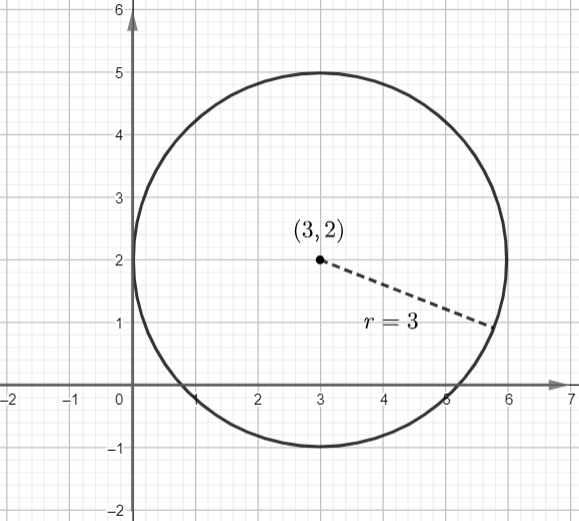Explanation: The given figure has the center as 3,2 and radius as r=3 units.
Now, let us plug those values, we get.

Question 4: Identify the center and radius of the circle, and sketch its graph:

(x+1)2+(y-2)= 16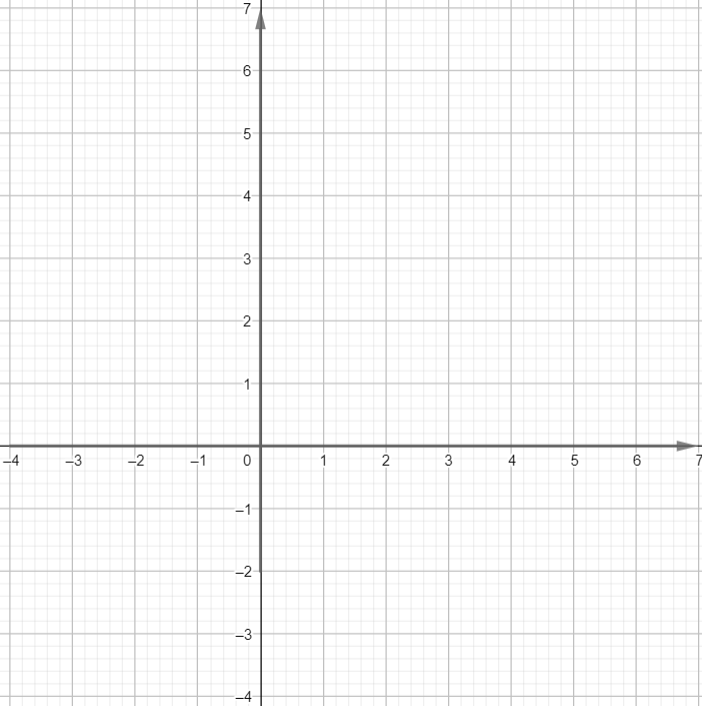Answer: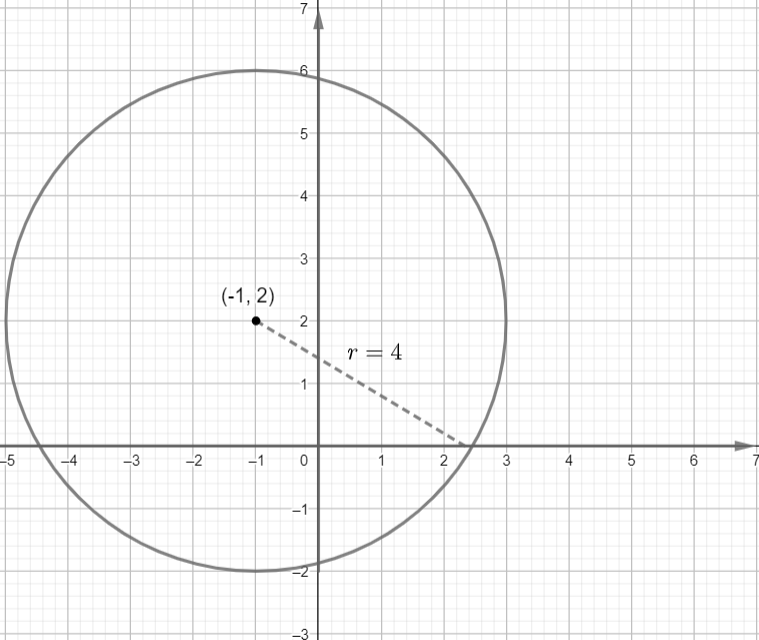Explanation: Use the formula of standard form of the equation of a circle.

Question 5: Translate the following equation of circle by 4 units right, 2 units down  (x+3)2+(y-5)= 25.

Explanation: Translate the 4 units down and then translate 2 units down.

Question 6: Translate the following equation of circle by 3 units left, 5 units up  (x+3)2+(y-5)= 64.

Explanation: Translate the 3 units down and then translate 5 units up.

Question 7: Identify the center and radius of the circle, and sketch its graph:

(x-2)2+(y+3)= 9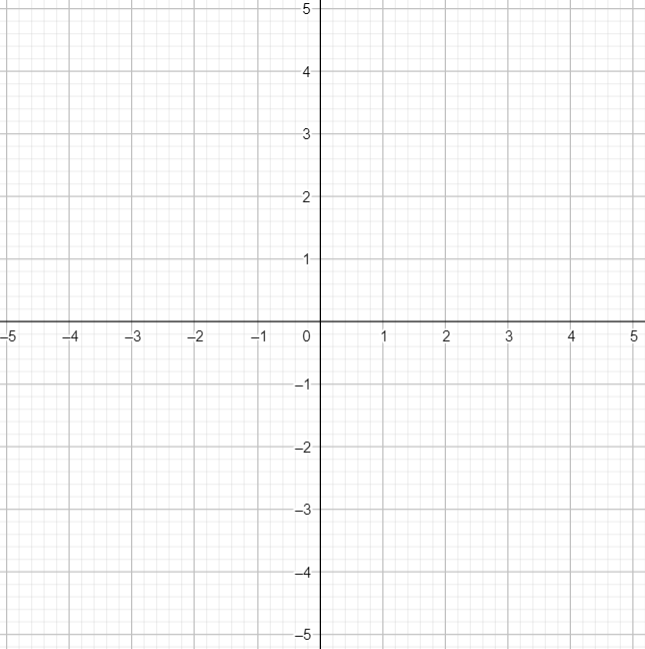Answer: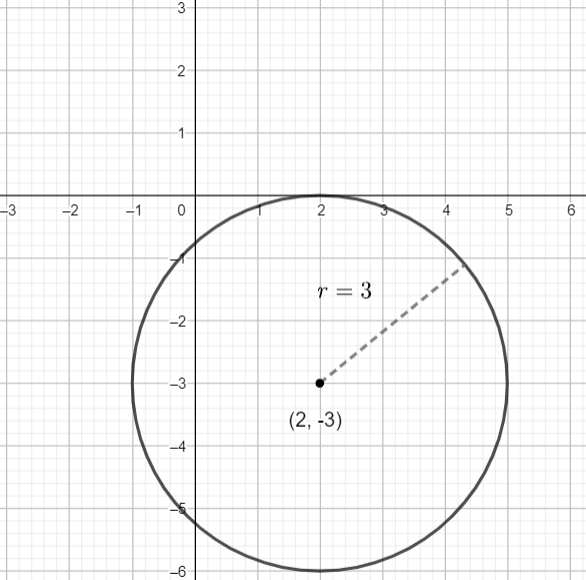Center is at 2,-3 and radius as r=3 units

Explanation: Use the formula of standard form of the equation of a circle.

Question 8: Write the following equation of the circle in standard form

x2+ 8x - 2y = 64 - y2

Explanation: Rewrite the equation and complete the square.

Question 9: Write the following equation of the circle in polar form.

x2+ 7x + y2 - 5y = 0

Explanation: Plug x+ y= r2, and x=rcosθ, y=rsinθ

Question 10: Write the following equation of the circle in polar form.

x- 9x + y2 + 5y = 0